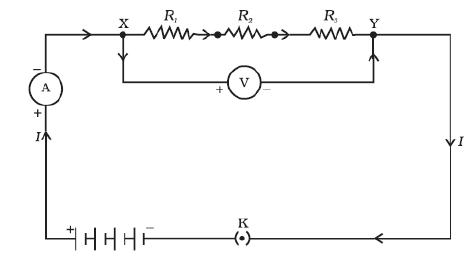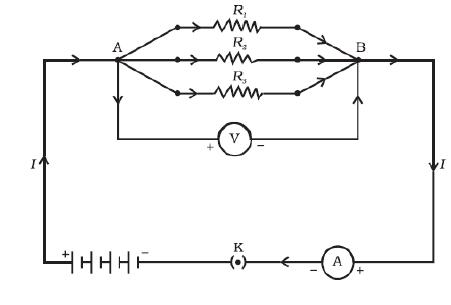## RESISTANCE OF A COMBINATION OF RESISTORS

There are two ways of combining the resistors in a circuit. Figure below shows an electric circuit in which three resistors having resistances R1, R2 and R3, respectively, are joined end to end. Here the resistors are said to be connected in series. In series, there is only one path for flow of current.Next Figure shows a combination of resistors in which three resistors are connected together between points X and Y. Here, the resistors are said to be connected in parallel. In parallel, there is separate path for flow of current in each resistor.## Resistors in Series

In a series combination of resistors
(1) Same current I flow through each resistor.
(2) Potential difference across each resistor is different. V1 across R1, V2 across R2 and Vacross R3.
(3) Total potential difference across the combination is equal to the sum of potential difference across the individual resistors. That is,
$V=V_1+V_2+V_3$

## Equivalent Resistance of Series CombinationLet I be the current through the circuit. The current through each resistor is also I. Applying the Ohm’s law to three resistors separately, we have
$V_1=IR_1$
$V_2=IR_2$
$V_3=IR_3$

Since
$V=V_1+V_2+V_3$

We have
$V=IR_1+IR_2+IR_3$
$V=I(R_1+R_2+R_3)$
$\frac{V}{I}=R_1+R_2+R_3$
or
$R_s=R_1+R_2+R_3$

Where, RS is the equivalent resistance of the series combination.

We can conclude that when several resistors are joined in series, the resistance of the combination RS equals the sum of their individual resistances, R1, R2, R3, and is thus greater than any individual resistance.

We can imagine a single resistor Rreplacing the three resistors joined in series such that the potential difference V across it, and the current I through the circuit remains the same.

## Resistors in Parallel

In parallel combination of resistors

(1) Potential difference across each resistor is same.
(2) Current through each resistor is different. I1 across R1, I2 across R2 and I3 across R3
(3) The total current I, is equal to the sum of the
separate currents through each resistor of the
combination.
$I=I_1+I_2+I_3$

## Equivalent resistance in parallel combinationOn applying Ohm’s law to each resistor of parallel combination, we have
$I_1=\frac{V}{R_1}$
$I_2=\frac{V}{R_2}$
$I_3=\frac{V}{R_3}$
Since
$I=I_1+I_2+I_3$

We have
$I=\frac{V}{R_1}+\frac{V}{R_2}+\frac{V}{R_3}$

$I=V(\frac{1}{R_1}+\frac{1}{R_2}+\frac{1}{R_3})$
$\frac{I}{V}=\frac{1}{R_1}+\frac{1}{R_2}+\frac{1}{R_3}$
$\frac{1}{R_P}=\frac{1}{R_1}+\frac{1}{R_2}+\frac{1}{R_3}$

Thus, we may conclude that the reciprocal of the equivalent resistance of a group of resistances joined in parallel is equal to the sum of the reciprocals of the individual resistances.

## Preference of parallel combination over series

We prefer parallel combination over series combination in domestic circuit because of the following reasons:

(1) In a series circuit the current is constant throughout the electric circuit. Thus it is impracticable to connect different appliances such as an electric bulb and an electric heater in series, because they need currents of different values to function properly.
(2) Another major disadvantage of a series circuit is that when one electrical device fails the circuit is broken and none of the devices connected in the circuit works.

On the other hand in parallel circuit...

(1) In a parallel circuit, different appliances are connected in different branches and each appliance gets its required amount of current in that branch.
(2) In a parallel circuit, if one component fails, the others are not affected.

## Other topic on this chapter: 1.   Electric Current. 2.   Electric Potential 3.   Ohm's Law 4.   Resistance and resistivity 5.   Combinations of resistors 6.   Heating effect of electric current 7.   Electric power

1. A wire of resistance R is cut into five equal parts. These five parts are then connected in parallel. If the equivalent resistance of this combination is R', then calculate the ratio R/R'.

2. What is the (a) highest and (b) lowest, total resistance that can be obtained by combining four resistors of values 4Ω, 8Ω, 12Ω and 24Ω?

3. How can three resistors of resistances 2Ω, 3Ω and 6Ω be connected to give a total resistance of (a) 4Ω (b) 1Ω ?

4. Two resistors with resistances 5Ω and 10Ω respectively are to be connected to a battery of 6V so as to obtain (i) minimum current flowing (ii) maximum current flowing

(a) How would you connect the resistance in each case?
(b) Calculate the strength of the total current in the circuit in the two cases.

5. A battery of 9V is applied across resistors of 0.2Ω, 0.3Ω, 0.4Ω, 0.5Ω and 12Ω connected in series. How much current would flow through the 12Ω resistors?

6. How many 176 Ω resistors (in parallel) are required to carry 5 A on a 220 V line?

7. A hot plate of an electric oven connected to a 220 V line has two resistance coils A and B, each of 24 Ω resistance, which may be used separately, in series, or in parallel. What are the currents in the three cases?

8. In the given figure R1 = 10 Ω, R2 = 40 Ω, R3 = 30 Ω, R4 = 20 Ω, R5 = 60 Ω, and a 12 V battery is connected to the arrangement.
Calculate (a) the total resistance in the circuit, and (b) the total current flowing in the circuit.

9. An electric lamp, whose resistance is 20 Ω, and a conductor of 4 Ω resistance are connected in series to a 6 V battery. Calculate (a) the total resistance of the circuit, (b) the current through the circuit, and (c) the potential difference across the electric lamp and conductor.

10. An electric lamp of 100 Ω, a toaster of resistance 50 Ω, and a water filter of resistance 500 Ω are connected in parallel to a 220 V source. What is the resistance of an electric iron connected to the same source that takes as much current as all three appliances, and what is the current through it?

11. Three resistors of resistances 5Ω, 10Ω and 30Ω are connected in parallel across a 12V battery. Calculate:
(a) Total resistance in the circuit.
(b) Total current in the circuit.
(c) Current through each resistor.